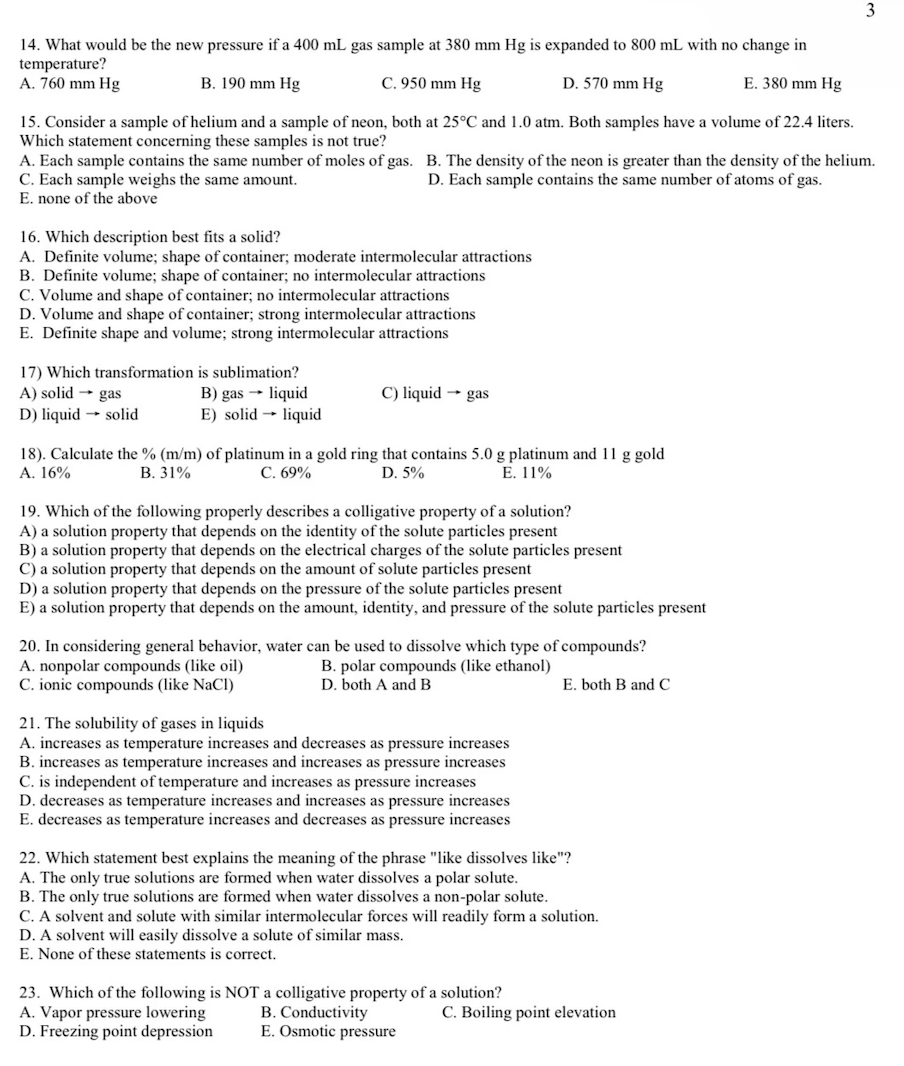Home / Expert Answers / Chemistry / 14-what-would-be-the-new-pressure-if-a-400ml-gas-sample-at-380mmhg-is-expanded-to-800ml-with-pa121

# (Solved): 14. What would be the new pressure if a 400mL gas sample at 380mmHg is expanded to 800mL with ...14. What would be the new pressure if a gas sample at is expanded to with no change in temperature? A. B. C. D. E. 15. Consider a sample of helium and a sample of neon, both at and . Both samples have a volume of 22.4 liters. Which statement concerning these samples is not true? A. Each sample contains the same number of moles of gas. B. The density of the neon is greater than the density of the helium. C. Each sample weighs the same amount. D. Each sample contains the same number of atoms of gas. E. none of the above 16. Which description best fits a solid? A. Definite volume; shape of container; moderate intermolecular attractions B. Definite volume; shape of container; no intermolecular attractions C. Volume and shape of container; no intermolecular attractions D. Volume and shape of container; strong intermolecular attractions E. Definite shape and volume; strong intermolecular attractions 17) Which transformation is sublimation? A) solid gas B) gas liquid C) liquid gas D) liquid solid E) solid liquid 18). Calculate the of platinum in a gold ring that contains platinum and gold A. B. C. D. E. 19. Which of the following properly describes a colligative property of a solution? A) a solution property that depends on the identity of the solute particles present B) a solution property that depends on the electrical charges of the solute particles present C) a solution property that depends on the amount of solute particles present D) a solution property that depends on the pressure of the solute particles present E) a solution property that depends on the amount, identity, and pressure of the solute particles present 20. In considering general behavior, water can be used to dissolve which type of compounds? A. nonpolar compounds (like oil) B. polar compounds (like ethanol) C. ionic compounds (like ) D. both and E. both and 21. The solubility of gases in liquids A. increases as temperature increases and decreases as pressure increases B. increases as temperature increases and increases as pressure increases C. is independent of temperature and increases as pressure increases D. decreases as temperature increases and increases as pressure increases E. decreases as temperature increases and decreases as pressure increases 22. Which statement best explains the meaning of the phrase "like dissolves like"? A. The only true solutions are formed when water dissolves a polar solute. B. The only true solutions are formed when water dissolves a non-polar solute. C. A solvent and solute with similar intermolecular forces will readily form a solution. D. A solvent will easily dissolve a solute of similar mass. E. None of these statements is correct. 23. Which of the following is NOT a colligative property of a solution? A. Vapor pressure lowering B. Conductivity C. Boiling point elevation D. Freezing point depression E. Osmotic pressure

We have an Answer from Expert